﻿ 9iceunity » Forum | Neco 2018 Mathematics Obj And Theory Answers – June/July Expo

Hi, Guest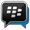AWOOF:- New MTN/ETISALAT/AIRTEL Unlimited Free Call/Browsing Code Now Available, Join Our BBM Channel Asap to Get it CLICK HERE (C0022EF41)JAMB 2019 KUNLESSI ANSWERS :-To Get Latest Answers About JAMB/WAEC/NECO/JUPEB 2019 ANSWERS, Kindly Click Here To join Us On WhatsApp Group (100% sure)
Forum » JAMB, WAEC, NECO PORTAL
Neco 2018 Mathematics Obj And Theory Answers – June/July Expo
Views: 3528  |  Comments: 4 |  Posted: 11:54 Fri, 01 Jun 2018♦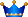Kunlessi (¥ 15734 NU) Star:UltimateCreated Topics: 1540 Replies: 110
Posted on: 11:54 Fri, 01 Jun 2018+++++++++++++++++++++++++++++++++++
Monday 4th June 2018
OBJ – General Mathematics – 10:00am – 11:45am
Essay – General Mathematics – 12:00noon –2:30pm++++++++++++++++++++++++++++++++++

********************************
Tell me How do you want to pass by waiting for Free Answers when those that Paid for The runz has finished, You are Just Starting.
********************************

MATHS OBJ:-
1-10: CDAAEABAEC
11-20:ACDDCDCDAC
21-30: CEBDEDCBBC
31-40: CBEECCBDCC
41-50: DDCBCDDBBA
51-60:BCEDCBBCEE
================

MATHS SYMBOLS
/ means DIVISION
* means Multiplication
tita You Should Know that.
rais means Raise to power or
^ means Raise to power
sqr rut/square rut means Square root
—–(1) means Equation 1
yr means Year

(1)
1a)
Log 10(20*-10)-log10(*+3)=log105
(20*-10/*+3)=log10 =5
20*-10/*+3=5
5(*+3)=20*-10
5*+15=20*-10
15+10=20*-5*
25=15*
*=25/15
*=5/3=1 2/3

1b)
Discount percent =15%
Discount amount =#600
Actual amount paid on the article =?
Original amount on the article =*
15%*=#600
15/100* =600
15*=600*100
15*=60000
*=60000/15
*=#4,000
Therefore actual amount paid on the article
=#4,000-#600
=#3,400
Actual amount paid on the article =#3,400

===============================

(2)

(2a)
(X^2 Y^-3 Z)^3/4/X^-1 Y^4 Z^5
= (X^2)^3/4/X^-1 * (Y^-3)^3/4/Y^4 * Z^3/4/Z^5
= X^3/2/X^-1 * Y^-9/4/Y^4 * Z^3/4/Z^5
=X^3/2+1 * Y^-9/4-4 * Z^3/4-5
=X^5/2 * Y^-25/4 * Z^-17/4
=X^10/4 * Y^-25/4 * Z^-17/4

=(X^10/Y^25 Z^17)^1/4

(2b)
√2/k + √2 = 1/k - √2
Multiply both sides by (k+√2)(k-√2)
√2(k-√2) = k+√2
√2k-√2 = k+√2
√2k-k = 2+√2
K(√2 -1) = 2+√2
K = 2+√2/√2-1
K = -(2+√2)/1-√2
Rationalizing
K = -(2+√2) * 1+√2/1-√2
K = -(2+√2)(1+√2)/1 - 2
K = (2+√2)(1+√2)
K = 2+2√2 + √2+2
K = 4+3√2

==================================
(3)
V = Mg√1 - r²
Square both sides
V² = m²g²(1-r²)
V²/m²g² = 1-r²
r² = 1 - v²/m²g²
r = √1-(v/mg)²
If v = 15, m = 20, and g = 10
r = √1 - (15/20*10)²
r = √1 - (0.075)²
r= √(1.075)(0.925)
r = √0.994375
r = 0.9972

===============================

No 4(i)
length of Arc of the sector
Titter= 72?, r = 14cm
L= titter / 360 x 2 pie r
==> L= 72/360 x 2 x 22/7 x 14
=44352/2520 = 17.6cm

(ii) perimeter of the sector
Perimeter = titter/360 x 2 pie r + 2r = 17.6 +(2x14) =17.6+28= 45.6cm

(iii) Area of the sector
Area = Titter/360 x pie r? =
72/360 x 22/7 x (14)? = 72 x 22 x 196/2520
Area= 310464/2520 = 123.2cm?

==================================
5a)
Mode = mass with highest frequency = 35kg
Median is the 18th mass
= 40kg.

(5b)
In a tabular form

Under Masses(x kg)
30,35,40,45,50,55

Under frequency(f)
5,9,7,6,4,4
Ef = 35

Under X-A
-10, -5, 0, 5, 10, 15

Under F(X-A)
-50, -45, 0, 30, 40, 60
Ef(X - A) = 35

Mean = A + (Ef(X - A)/Ef)
= 40 + 35/35
= 40 + 1
= 41kg

==================================

(6)

==================================

(7ai)
T3=>a+2d=6(eqi)
T7=>a+6d=30(eqii)
Eqii minus eqi gives
6d-2d=30-6
4d=24
d=24/4
d=6
Common difference=6

(7aii)
Putting d=6 into eqi
a+2(6)=6
a+12=6
a=6-12
a=-6
(7aiii)
10th term T10=a+9d
=-6+9(6)
=-6+54
=48

(7bi)
T3=>ar²=9/2(eqi)
T6=>ar^5=243/16(eqii)
Dividing eqii by eqi
ar^5/ar²=243/16 divided by 9/2
r³=243/16*2/9
r³=27/8
r³=3³/2³
r=3/2
Putting this into eqi
a(3/2)²=9/2
a(9/4)=9/2
a=9/2*4/9
a=4/2=2

(7bii)
Common ratio r=3/2 as above

==================================

(8.)
x=a+by(eqi)
when y=5 and x=19
19=a+5b(eqii)
when y=10 and x=34
34=a+10b(eqiii)
solving eqii and eqiii
a+10b=34
a+5b=19
=>5b=15
b=15/5=3
putting b=3 in eqii
19=a+5(3)
19=a+15
a=19-15
a=4

(8i)
Putting a=4 and b=3 in eqi
x=4+3y
This is the relationship between xand y

(8ii)
When y=7
x=4+3(7)
x=4+21
x=25

(8b)
3x/x+2 - 5x/3x - 1 + 1/3

Find the L. C. M

3(3x-1)(3x)-3(x+2)(5x)+(x+2)(3x-1)/(x+2)(3x-1)(3)
27x²-9x-15x²-30x+3x²-x+6x-2/3(x+2)(3x-1)

Collect like terms

15x²-34x-2/3(x+2)(3x-1

===============================

(9a)

==================================

(10a)
Obtuse 105 + reflex Reflex =255°
Now 2w = reflex 2w =255°
W = 255/2 =127.5°

Also 2x = obtuse 2x = 105°
X = 105/2 = 52.5°
Now EDF = y(base angles of an isosceles triangle)
BED=X=52.5°(angles in the same segment)
EFD+EDF=BED (sum of interior angles of a triangle equal exterior angle)
Y+y = 52.5°
2y = 52.5°
Y = 52.5°/2
=26.25°

(10b)
Draw the diagram
|TB|/|BR| = TanR
100/|BR| = Tan60°
|BR| = 100/tan60
|BR| = 100√3
|BR| = 100√3 * √3/√3
=100√3/3m OR 57.7m

==================================
(11a)
x+y/2 =11
x+y= 11*2
x+y= 22 ---(1)
x-y= 4 ----(11)
x+y = 22----(1)
-
x-y= 4----(11)
____________
2y = 18
y= 18/2
y=9
Substitute y=9 in equ 1
x+9=22
x=22-9
x=13
x=13, y=9
x+y= 13+9= 22
Sum of the two number

(11b)
(6x + 3) dx
integrating
6x²/2+3x/1

6
= {3x²+3x}
1
= 6
{3x²+3x}
1

= [3(6)²+3(6)] - [3(¹)²+³(1)]

= [3(36)+18] - [3+4]
= [108+18] - 
= 126-9
= 117

(11c)
y = x² + 5x - 3 (x = 2)
y = 2² + 5(2) - 3
y = 4 + 10 - 3
y = 14 - 3
y = 11
Gradient of the curve = 11

==================================

(12)

(12a)
Pr of Abu to pass = 3/7
Pr of Abu to fail = 1 - 3/7 = 7-3/7 = 4/7

Pr of kuranku to pass = 5/9
Pr of kuranku to fail = 1 - 5/9 = 9 - 5/9 = 4/9

Pr of musa to pass = 12/13
Pr of musa to fail = 1 - 12/13 = 13 - 12/13 = 1/13

Pr of only one of them passing is
=(3/7*4/9*1/13)+(5/9*4/7*1/13)+(12/13*4/7*4/9)
=12/819+ 20/819 + 192/819
=12+20+192/819 = 224/819
= 32/117

(12b)
10Red + 8green + 7blue = 25

(i)
pr of different colour is
Prof(RG)+(RB)+(GB)+(BG)+(BR) +(GR)
=(10/25*8/24)+(10/25*7/24)+(8/25*7/24)+(7/25*8/24)+(7/25*10/24)+(8/25*10/24)
=80/100 + 70/600 + 56/600 + 56/600 + 70/600 + 80/600
= 80+70+56+56+70+80/600
= 412/800 = 103/200

(ii)
pr of atleast one must be
=Pr[RB+BR+GB+BG+BB]
= (10/25*7/24)+(7/25*10/24)+(8/25*7/24)+ (7/25*8/24) + (7/25*7/24)
=70/600+70/600+56/600+56/600+49/600
=70+70+56+56+49
/600
=301/600

================
COMPLETED
================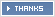:- Ifadare, Ifadare, Emeka Obih, Emeka Obih,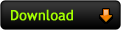You Might Also Like This:-

Please dont forget to click the share button

Replies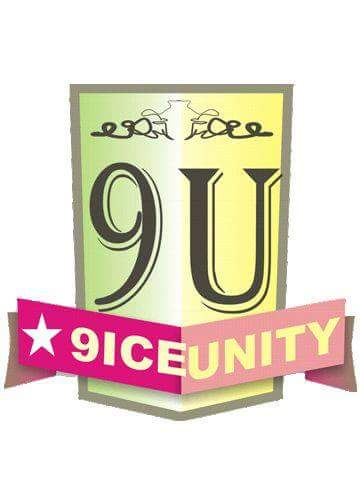♦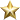Ifadare (¥ 9 NU) Star: Novice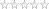Created Topics: 0 Replies: 3
Posted on: 11:54 Fri, 01 Jun 2018
I will send the Mtn card to You Soon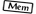♦Ifadare (¥ 9 NU) Star: NoviceCreated Topics: 0 Replies: 3
Posted on: 11:54 Fri, 01 Jun 2018
I have sent it oo♦Emeka Obih (¥ 6 NU) Star: NoviceCreated Topics: 0 Replies: 2
Posted on: 11:54 Fri, 01 Jun 2018
I love ur website Baba help me today♦Emeka Obih (¥ 6 NU) Star: NoviceCreated Topics: 0 Replies: 2
Posted on: 11:54 Fri, 01 Jun 2018
I will chart with u on WhatsApp